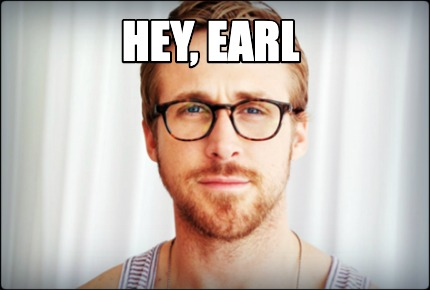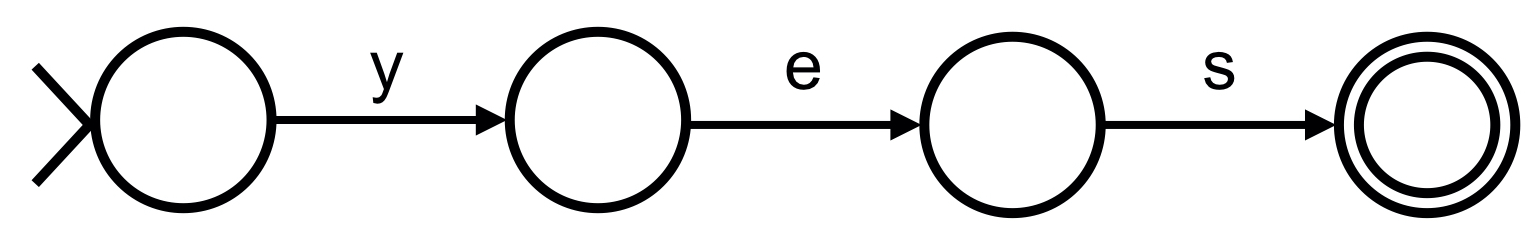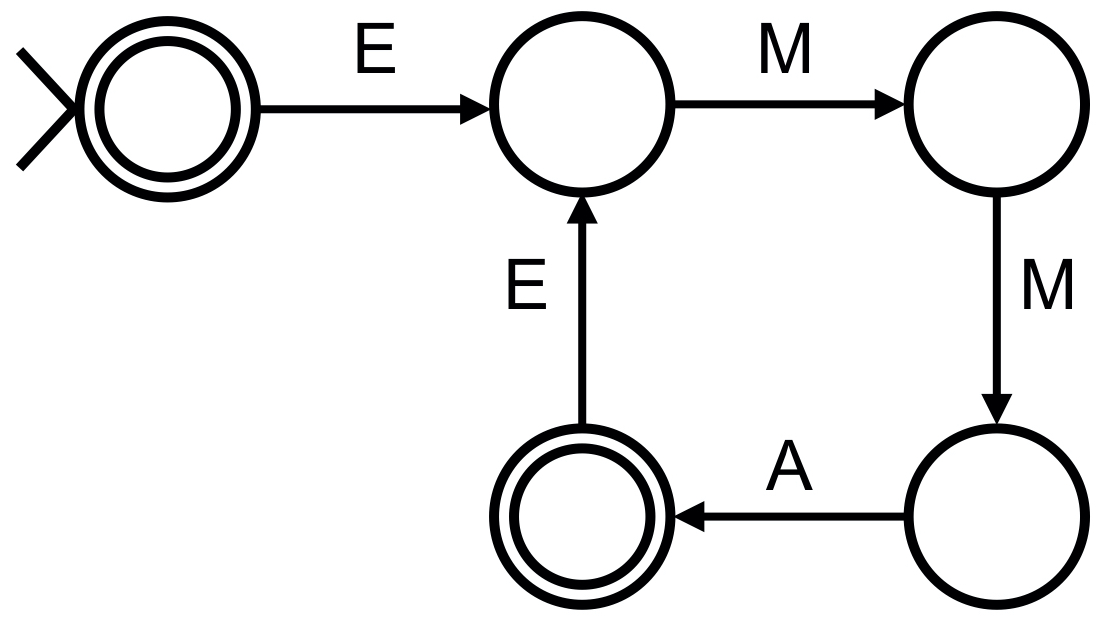# Hey, EarlRecall that a “regular expression,” otherwise known as a “regex,” is a pattern designed to match some string. For instance, a regex like `y` would match any string containing a `y`, a regex like `yes` would match any string containing `yes`, a regex like `^yes` would match any string starting with `yes`, and a regex like `^yes\$` would match only the string `yes`.

Read up on regular expressions via Python’s Regular Expression HOWTO, focusing on these sections specifically:

1. (1 point.) Describe, in a sentence, the set of strings that a regex like `^yes+\$` would match.

2. (1 point.) Describe, in a sentence, the set of strings that a regex like `^yes\s*\$` would match.

3. (2 points.) Consider the Python program below.

`````` import re
words = input("Say something!\n")
p = re.compile("my name is (.*)", re.IGNORECASE)
matches = p.search(words)
if matches:
print(f"Hey, {matches}.")
else:
print("Hey, you.")
``````

Given input like `My name is Earl`, this program politely outputs `Hey, Earl`. Given input like `My name is Earl Hickey`, though, this program outputs `Hey, Earl Hickey`, which feels a bit formal. Propose how to modify the argument to `re.compile` in such a way (without hardcoding `Earl`) that this program would instead output `Hey, Earl` in both cases.

## DFAs

It turns out that regular expressions can be implemented in software with “deterministic finite automata” (DFAs), otherwise known as “state machines.” DFAs can even be implemented with diagrams as well, wherein each node (i.e., circle) represents a “state” and each edge (i.e., line) represents a “transition.” For instance, below is a DFA that implements a regex like `^yes\$`:A DFA is a machine (or algorithm, really) in the sense that it takes some input and produces some output. Its input is a string (like `y` or `yes`). Its output is a decision: to “accept” or to “reject” that string. A DFA accepts a string if that string matches a pattern, the very regex that the DFA implements. A DFA rejects a string if that string doesn’t match the pattern.

How does a DFA decide whether its input matches a pattern? Well, when a DFA is “turned on,” so to speak, it’s considered to be in its “start” state, as is indicated by a circle with a >. It then looks at the first character of its input. If there is an edge labeled with that same character from the start state to some other state, the DFA transitions from its start state to the other, thereby consuming the character. The DFA then looks at the second character of its output. If there is an edge labeled with that same character from its current state to another, it transitions again, consuming the character. If, upon consuming every character of its input, the DFA finds itself in an “accept” state, as is indicated by a circle within a circle, it must be the case that the string matched the pattern, and so the DFA accepts it (and turns off). If, though, at any point, the DFA finds itself in a “reject” state, as is indicated with a circle without a circle within it, having consumed all of its input or unable to transition from one state to another because there’s no edge labeled the same as the character currently being read, it must be the case that the input didn’t match the pattern, and so the DFA rejects it (and turns off).

Consider, for instance, how the DFA above might process an input like `y`, which doesn’t match `^yes\$`.

1. The DFA starts in its start state.
2. The DFA reads the first (and only) character of its input, `y`. Because there is an edge labeled `y`, the DFA transitions from its start state to its second state, thereby consuming that `y`.
3. Because the DFA has consumed all of its input but is in a reject state, the DFA rejects `y`, just as it should, because `y` doesn’t match `^yes\$`.

Now consider how the DFA above might process an input like `yes`, which does match `^yes\$`:

1. The DFA starts in its start state.
2. The DFA reads the first character of its input, `y`. Because there is an edge labeled `y`, the DFA transitions from its start state to its second state, thereby consuming that `y`.
3. The DFA reads the second character of its input, `e`. Because there is an edge labeled `e`, the DFA transitions from its second state to its third state, thereby consuming that `e`.
4. The DFA reads the third (and final) character of its input, `s`. Because there is an edge labeled `s`, the DFA transitions from its third state to its fourth state, thereby consuming that `s`.
5. Because the DFA has consumed all of its input and is in an accept state, the DFA accepts `yes`, just as it should, because `yes` matches `^yes\$`.

Consider the DFA below.1. (2 points.) What regex does this DFA implement?

2. (3 points.) What are three strings that this DFA would accept?

3. (1 point.) What is one string that this DFA would not accept?